Question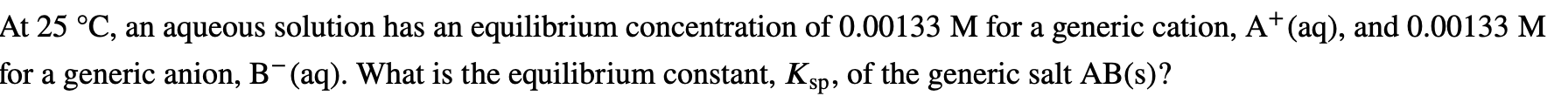AB(s)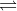A+(aq)    +    B-(aq)

Equilibrium concentration (M)                                       0.00133 M        0.00133 M

Equilibrium constant,

Ksp = [A+][B+]

= (0.00133)(0.00133)

= 1.77 x 10-6

Hence, the equilibrium constant = 1.77 x 10-6

#### Earn Coins

Coins can be redeemed for fabulous gifts.

Similar Homework Help Questions
• ### At 25 °C, an aqueous solution has an equilibrium concentration of 0.00489 M for a generic...

At 25 °C, an aqueous solution has an equilibrium concentration of 0.00489 M for a generic cation, A (aq), and 0.00245 M for a generic anion, B2–(aq). What is the equilibrium constant, Ksp, of the generic salt A2B(s)?

• ### At 25 °C, an aqueous solution has an equilibrium concentration of 0.00415 M for a generic cation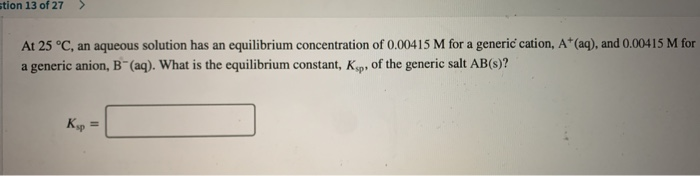At 25 °C, an aqueous solution has an equilibrium concentration of 0.00415 M for a generic cation, A+ (aq), and 0.00415 M for a generic anion, B" (aq). What is the equilibrium constant, Kop, of the generic salt AB(s)?

• ### At 25 °C, an aqueous solution has an equilibrium concentration of 0.00177 M for a generic...

At 25 °C, an aqueous solution has an equilibrium concentration of 0.00177 M for a generic cation, A 2 + ( aq ) , and 0.00354 M for a generic anion, B − ( aq ) . What is the equilibrium constant, K sp , of the generic salt AB 2 ( s ) ? K sp =

• ### At 25 °C, an aqueous solution has an equilibrium concentration of 0.00427 M for a generic...

At 25 °C, an aqueous solution has an equilibrium concentration of 0.00427 M for a generic cation, A+(aq), and 0.00214 M for a generic anion, B2−(aq). What is the equilibrium constant, ?sp, of the generic salt A2B(s)?

• ### At 25 °C, an aqueous solution has an equilibrium concentration of 0.00161 M for a generic...

At 25 °C, an aqueous solution has an equilibrium concentration of 0.00161 M for a generic cation, A + ( aq ) , and 0.000805 M for a generic anion, B 2 − ( aq ) . What is the equilibrium constant, K sp , of the generic salt A 2 B ( s ) ? K sp =

• ### At 25 °C, only 0.0450 mol of the generic salt AB is soluble in 1.00 L...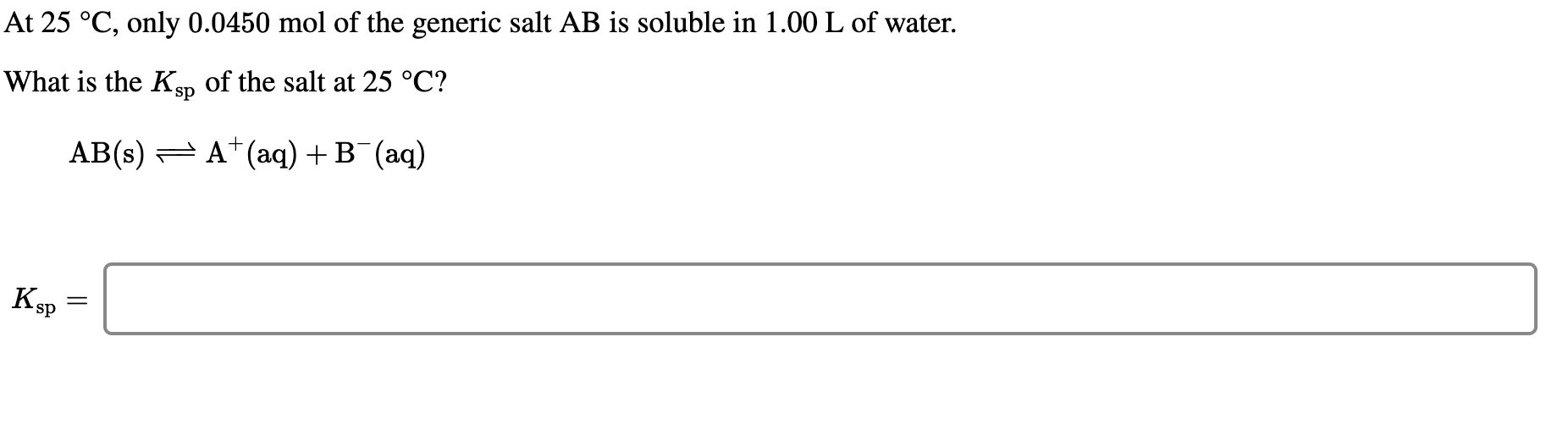At 25 °C, only 0.0450 mol of the generic salt AB is soluble in 1.00 L of water. What is the Ksp of the salt at 25 °C? AB(s) 7 A+ (aq) +B+ (aq) Ksp = At 22 °C, an excess amount of a generic metal hydroxide, M(OH)2, is mixed with pure water. The resulting equilibrium solution has a pH of 10.18. What is the Ksp of the salt at 22 °C? ot a generic metal hydroxide, M(OH), is mixed...

• ### The generic metal A forms an insoluble salt AB(s) and a complex AC5(aq). The equilibrium concentrations in a solution of...

The generic metal A forms an insoluble salt AB(s) and a complex AC5(aq). The equilibrium concentrations in a solution of AC5 were found to be [A]=0.100 M[A]=0.100 M, [C]=0.0260 M[C]=0.0260 M, and [AC5]=0.100 M[AC5]=0.100 M. Determine the formation constant, Kf, of AC5. ?f= The solubility of AB(s) in a 1.000 M solution of C(aq) is found to be 0.1310.131 M. What is the Ksp of AB? ?sp=

• ### The generic metal A forms an insoluble salt AB(s) and a complex AC5(aq). The equilibrium concentrations...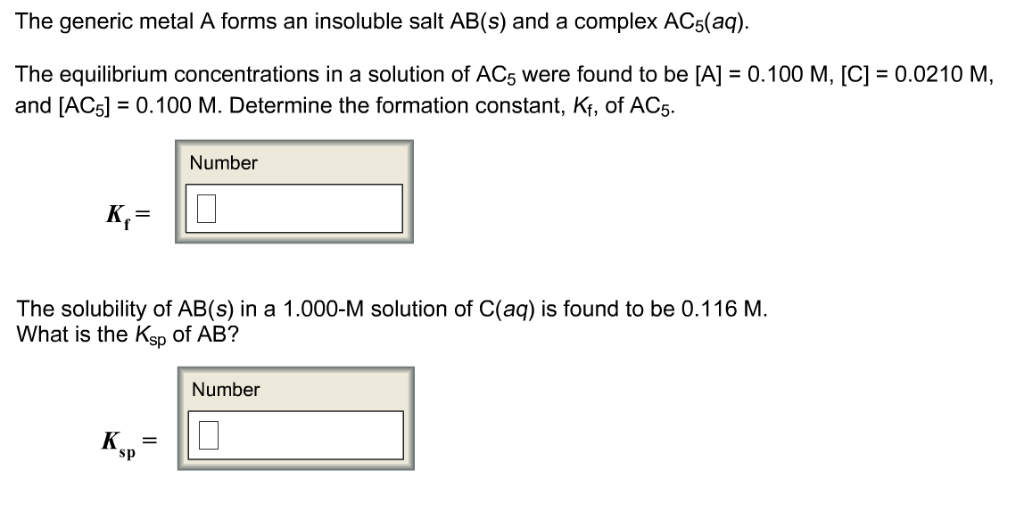The generic metal A forms an insoluble salt AB(s) and a complex AC5(aq). The equilibrium concentrations in a solution of AC5 were found to be [A] 0.100 M, [C] 0.0210 M, and [ACSl0.100 M. Determine the formation constant, K, of AC5 Number The solubility of AB(s) in a 1.000-M solution of C(aq) is found to be 0.116 M. What is the Ksp of AB? Number sp

• ### At 25 °C, only 0.0450 mol of the generic salt AB is soluble in 1.00 L...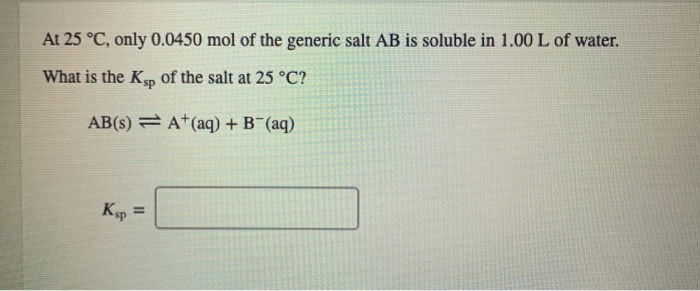At 25 °C, only 0.0450 mol of the generic salt AB is soluble in 1.00 L of water. What is the Ksp of the salt at 25 °C? AB(8) = A+(aq) + B+ (aq) Ksp =

• ### At 25 °C, only 0.0650 mol of the generic salt AB3 is soluble in 1.00 L...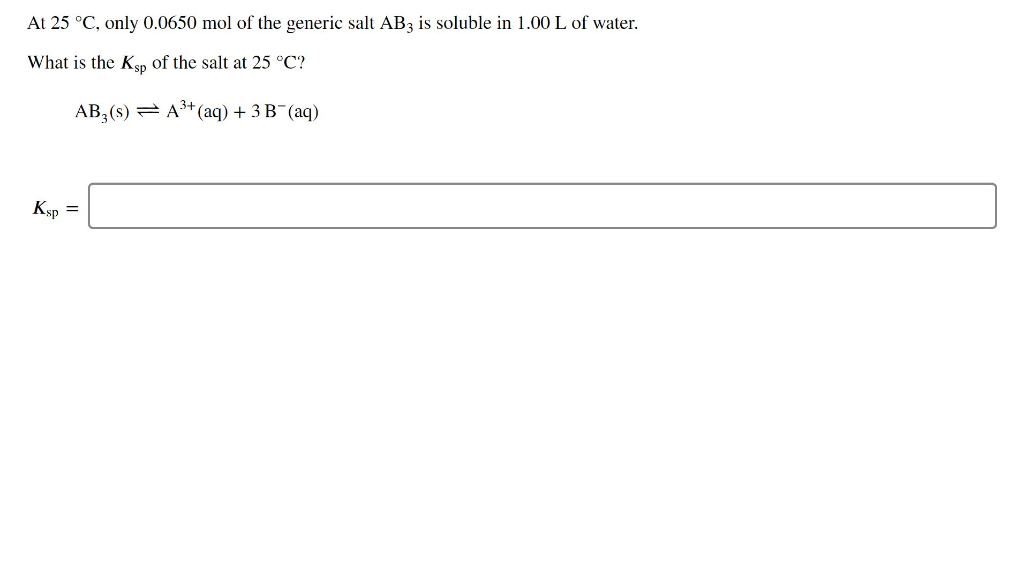At 25 °C, only 0.0650 mol of the generic salt AB3 is soluble in 1.00 L of water. What is the Ksp of the salt at 25 °C? AB,(S) = A+ (aq) + 3B (aq) Ksp =